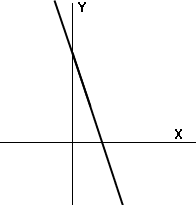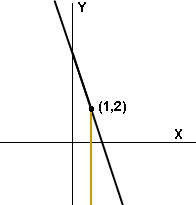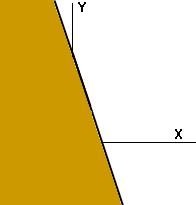I am a secondary algebra I student

How do I find x and y and graph this problem.

3x + y < 5

Lori

Hi Lori
 First add -3x to both sides to rewrite your inequality as y < 5 - 3x. Now consider the equation y = 5 - 3x an plot its graph.Choose an x-value, for example x = 1. The point satisfying y = 5 - 3x with x = 1 is (1,2). The points satisfying y < 5 - 3x with x = 1 are the points with x = 1 and y value less than 2, that is the points in the diagram to the right coloured orange.Do the same for every x-value to see that the graph of y < 5 - 3x is the half-plane coloured orange in the diagram to the right.Cheers,
Penny
Go to Math Central